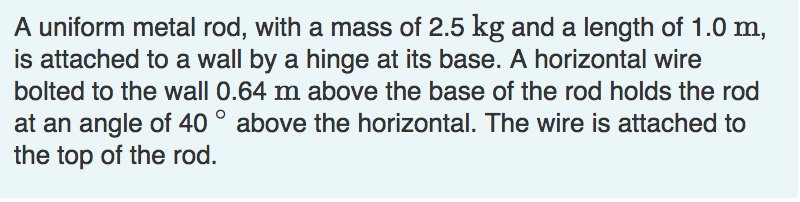# A uniform metal rod, with a mass of 2.5 kg and a length of 1.0 mis attached to a wall by a hinge at its base. A horizontal wireat an angle of 40° above the horizontal. The wire is attached tothe top of the rod.

Question

Find the tension in the wire, the horizontal component of the force exerted on the rod by the hinge, and the vertical component of the force exerted on the rod by the hinge.help_outlineImage TranscriptioncloseA uniform metal rod, with a mass of 2.5 kg and a length of 1.0 m is attached to a wall by a hinge at its base. A horizontal wire at an angle of 40° above the horizontal. The wire is attached to the top of the rod. fullscreen
check_circle

Step 1

Given: Mass of the uniform metal rod, m = 2.5 kg

Length of the metal rod, L = 1 m

The height of the supporting wire fixed from the hinged position of the beam on the wall, h = 0.64 m

Angle = 40o

Find: (a) Tension in the wire

(b) Horizontal component of force exerted on the rod by hinge

(c) Vertical component of force exerted on the rod by hinge

Step 2

Free body diagram of the beam is as shown:

Step 3

(a) Balancing the torque equation, a...

### Want to see the full answer?

See Solution

#### Want to see this answer and more?

Solutions are written by subject experts who are available 24/7. Questions are typically answered within 1 hour.*

See Solution
*Response times may vary by subject and question.
Tagged in

### Physics